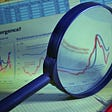# Chebyshev’s Inequality in Statistics — Explained

This article could be considered as an introduction for beginners or a quick refresher for experienced.

# What is Chebyshev’s Inequality?

Chebyshev’s theorem is used to determine the proportion of events you would expect to find within a certain number of standard deviations from the mean.

For normal distributions, about 68% of results will fall between +1 and -1 standard deviations from the mean. About 95% will fall between +2 and -2 standard deviations.

But what if our random variable is not in Normal distribution, and when they are not in Normal Distribution how do we find the number of events that lie under a certain number of standard deviations from the mean. It is the reason we use Chebyshev’s Inequality.

For a population or sample, the proportion of observations is no less than

(1 — (1 / k² ))

Here the value of K is the number of Standard Deviations from the mean. For example, let’s take the Second Standard deviation.

Then our k = 2

=> (1–1/4) => 3/4 => 0.75

This value of 0.75 means that more than 75% of events will lie under the second Standard Deviation.

# Conclusion

There is one thing that you should keep in mind before using Chebyshev’s Inequality which is “ The random variable must not be in Normal distribution”. If the variable is not in Normal distribution you can use Chebyshev’s Inequality and find greater than how many events like under a certain number of standard deviations from the mean.

--

--

--

## More from Adith - The Data Guy

Love podcasts or audiobooks? Learn on the go with our new app.

## The Logic of Risk Taking## Reversion to the mean? Still tough to profit from it## Who is W.D Gann?## Factors of 150## Understanding Proofs by Euclid — 101## Factors of 117## The Beauty of Moment Generating Functions## Probability vs. Likelihood## Phi Correlation Coefficient in R## The Assumptions in Linear Regression